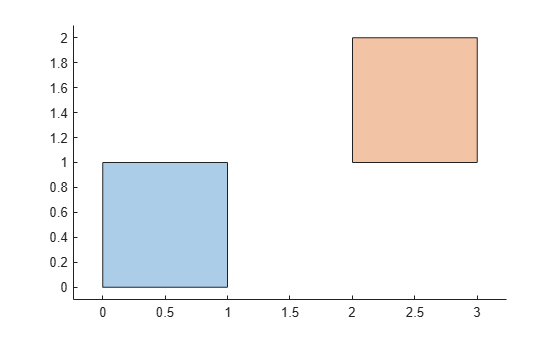# translate

Translate `polyshape`

## Syntax

``polyout = translate(polyin,v)``
``polyout = translate(polyin,x,y)``

## Description

example

````polyout = translate(polyin,v)` returns a `polyshape` object defined by translating `polyin` by `v`. The first element of `v` specifies the distance to translate in the x direction, and the second element specifies the distance to translate in the y direction.```
````polyout = translate(polyin,x,y)` specifies the x and y translation amounts as separate arguments.```

## Examples

collapse all

Create a square, then translate the square to the right by two units, and up by one unit. Plot both squares.

```polyin = polyshape([0 0 1 1],[1 0 0 1]); polyout = translate(polyin,[2 1]); plot([polyin polyout]) axis equal```## Input Arguments

collapse all

Input `polyshape`, specified as a scalar, vector, matrix, or multidimensional array.

Data Types: `polyshape`

Translation distance, specified as a two-element row vector. The first element of `v` specifies the distance to translate in the x direction, and the second element specifies the distance to translate in the y direction. Positive distances translate right and up, and negative distances translate left and down. When the input `polyshape` is an array, each element of the array is translated according to `v`.

Data Types: `double` | `single` | `int8` | `int16` | `int32` | `int64` | `uint8` | `uint16` | `uint32` | `uint64`

x translation distance, specified as a scalar. Positive distances translate right, and negative distances translate left. When the input `polyshape` is an array, each element of the array is translated in the x direction according to `x`.

Data Types: `double` | `single` | `int8` | `int16` | `int32` | `int64` | `uint8` | `uint16` | `uint32` | `uint64`

y translation distance, specified as a scalar. Positive distances translate up, and negative distances translate down. When the input `polyshape` is an array, each element of the array is translated in the y direction according to `y`.

Data Types: `double` | `single` | `int8` | `int16` | `int32` | `int64` | `uint8` | `uint16` | `uint32` | `uint64`